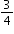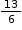# Standard #: MAFS.4.NF.2.4 (Archived Standard)

This document was generated on CPALMS - www.cpalms.org

Apply and extend previous understandings of multiplication to multiply a fraction by a whole number.
1. Understand a fraction a/b as a multiple of 1/b. For example, use a visual fraction model to represent 5/4 as the product 5 × (1/4), recording the conclusion by the equation 5/4 = 5 × (1/4).
2. Understand a multiple of a/b as a multiple of 1/b, and use this understanding to multiply a fraction by a whole number. For example, use a visual fraction model to express 3 × (2/5) as 6 × (1/5), recognizing this product as 6/5. (In general, n × (a/b) = (n × a)/b.)
3. Solve word problems involving multiplication of a fraction by a whole number, e.g., by using visual fraction models and equations to represent the problem. For example, if each person at a party will eat 3/8 of a pound of roast beef, and there will be 5 people at the party, how many pounds of roast beef will be needed? Between what two whole numbers does your answer lie?

### Remarks

Examples of Opportunities for In-Depth Focus

This standard represents an important step in the multi-grade progression for multiplication and division of fractions. Students extend their developing understanding of multiplication to multiply a fraction by a whole number.

### General Information

Subject Area: Mathematics
Domain-Subdomain: Number and Operations - Fractions
Cluster: Build fractions from unit fractions by applying and extending previous understandings of operations on whole numbers. (Major Cluster) -

Clusters should not be sorted from Major to Supporting and then taught in that order. To do so would strip the coherence of the mathematical ideas and miss the opportunity to enhance the major work of the grade with the supporting clusters.

Date of Last Rating: 02/14
Status: State Board Approved - Archived
Assessed: Yes

### Test Item Specifications

N/A

Assessment Limits :
Fractions may only be multiplied by a whole number. Denominators of given fractions are limited to: 2, 3, 4, 5, 6, 8, 10, 12, 100.
Calculator :

None

Context :

Allowable

### Sample Test Items (1)

 Test Item # Question Difficulty Type Sample Item 1 Daniella fills a container with soil by using a bowl. The bowl holdscup of soil. Daniella uses 13 full bowls of soil to fill the container.How many cups of soil does the container hold? N/A EE: Equation Editor

#### Related Courses

 Course Number1111 Course Title222 5012060: Mathematics - Grade Four (Specifically in versions: 2014 - 2015, 2015 - 2022, 2022 and beyond (current)) 7712050: Access Mathematics Grade 4 (Specifically in versions: 2014 - 2015, 2015 - 2018, 2018 - 2022, 2022 and beyond (current)) 5012065: Grade 4 Accelerated Mathematics (Specifically in versions: 2019 - 2022, 2022 and beyond (current)) 5012015: Foundational Skills in Mathematics 3-5 (Specifically in versions: 2019 - 2022, 2022 and beyond (current))

#### Educational Games

 Name Description Ice Ice Maybe: An Operations Estimation Game This fun and interactive game helps practice estimation skills, using various operations of choice, including addition, subtraction, multiplication, division, using decimals, fractions, and percents. Various levels of difficulty make this game appropriate for multiple age and ability levels. Addition/Subtraction: The addition and subtraction of whole numbers, the addition and subtraction of decimals. Multiplication/Division: The multiplication and addition of whole numbers. Percentages: Identify the percentage of a whole number. Fractions: Multiply and divide a whole number by a fraction, as well as apply properties of operations. Fraction Quiz Test your fraction skills by answering questions on this site. This quiz asks you to simplify fractions, convert fractions to decimals and percentages, and answer algebra questions involving fractions. You can even choose difficulty level, question types, and time limit.

#### Formative Assessments

 Name Description How Much Sugar? Students are asked to multiply a fraction by a whole number to solve a word problem and to represent the product with a visual fraction model. Training for a Race Students are asked to multiply an improper fraction by a whole number to solve a word problem and use a visual model or equation to represent the problem. How Many One Fourths? Students are asked to multiply a fraction by a whole number and to represent the product with a visual fraction model. Fractions and Multiples Students use a visual fraction model to explain how many one sixths are inand record their work with an equation.

#### Original Student Tutorials

 Name Description Buffy's Bakery Part 4- Multiplying a Fraction by a Whole: Standard Algorithm Help Buffy multiply fractions by whole numbers using the standard algorithm in addition to visual fraction models in this bakery-themed, interactive tutorial. This is part 4 of a 4-part series. Click below to open other tutorials in the series. Buffy’s Bakery Part 3: Using Models to Multiply a Fraction by a Whole Number Help Buffy the Baker multiply a fraction by a whole using models in this sweet interactive tutorial. This is part 3 of a 4-part series. Click below to open other tutorials in the series. Multiplying Fractions with Bake Sale Mania Find the total amounts of repeated fraction quantities by multiplying a fraction by a whole number using visual models that represent real-world problems and cookies in this interactive tutorial.

 Name Description Sugar in six cans of soda This task provides a familiar context allowing students to visualize multiplication of a fraction by a whole number. This task could form part of a very rich activity which includes studying soda can labels. Connor and Makayla Discuss Multiplication The purpose of this task is to have students think about the meaning of multiplying a number by a fraction, and use this burgeoning understanding of fraction multiplication to make sense of the commutative property of multiplication in the case of fractions.

#### Tutorial

 Name Description Multiplying a Fraction by a Whole Number In this Khan Academy video visual fraction models are used to represent the multiplication of a whole number times a fraction.

#### Original Student Tutorials

 Name Description Buffy's Bakery Part 4- Multiplying a Fraction by a Whole: Standard Algorithm: Help Buffy multiply fractions by whole numbers using the standard algorithm in addition to visual fraction models in this bakery-themed, interactive tutorial. This is part 4 of a 4-part series. Click below to open other tutorials in the series. Buffy’s Bakery Part 3: Using Models to Multiply a Fraction by a Whole Number: Help Buffy the Baker multiply a fraction by a whole using models in this sweet interactive tutorial. This is part 3 of a 4-part series. Click below to open other tutorials in the series. Multiplying Fractions with Bake Sale Mania: Find the total amounts of repeated fraction quantities by multiplying a fraction by a whole number using visual models that represent real-world problems and cookies in this interactive tutorial.

#### Educational Games

 Name Description Ice Ice Maybe: An Operations Estimation Game: This fun and interactive game helps practice estimation skills, using various operations of choice, including addition, subtraction, multiplication, division, using decimals, fractions, and percents. Various levels of difficulty make this game appropriate for multiple age and ability levels. Addition/Subtraction: The addition and subtraction of whole numbers, the addition and subtraction of decimals. Multiplication/Division: The multiplication and addition of whole numbers. Percentages: Identify the percentage of a whole number. Fractions: Multiply and divide a whole number by a fraction, as well as apply properties of operations. Fraction Quiz: Test your fraction skills by answering questions on this site. This quiz asks you to simplify fractions, convert fractions to decimals and percentages, and answer algebra questions involving fractions. You can even choose difficulty level, question types, and time limit.

 Name Description Sugar in six cans of soda: This task provides a familiar context allowing students to visualize multiplication of a fraction by a whole number. This task could form part of a very rich activity which includes studying soda can labels. Connor and Makayla Discuss Multiplication: The purpose of this task is to have students think about the meaning of multiplying a number by a fraction, and use this burgeoning understanding of fraction multiplication to make sense of the commutative property of multiplication in the case of fractions.

#### Tutorial

 Name Description Multiplying a Fraction by a Whole Number: In this Khan Academy video visual fraction models are used to represent the multiplication of a whole number times a fraction.